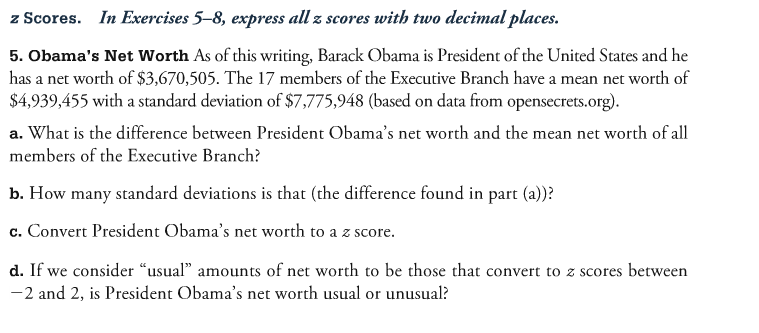# (SOLVED) What is the difference between President Obama's net worth and the mean net worth of all members of the Executive Branch?

Discipline: Mathematics

Paper Format: APA

Pages: 1 Words: 275

Question

Obama's Net Worth As of this writing, Barack Obama is President of the United States and he has a net worth of \$3, 670, 505. The 17 members of the Executive Branch have a mean net worth of \$4, 939, 455 with a standard deviation of \$7, 775, 948 (based on data from opensecrets.org). What is the difference between President Obama's net worth and the mean net worth of all members of the Executive Branch? How many standard deviations is that (the difference found in part (a))? Convert President Obama's net worth to a z score. If we consider "usual" amounts of net worth to be those that convert to 2 scores between - 2 and 2, is President Obama's net worth usual or unusual?Obama = 3670505

EB = 4939455 , sd = 7775948

a) The difference is 3670505 -4939455 =-1268590

b) as the Sd is 7775948 , and the difference is 1268590 ,where SD > Difference

so it lies within 1 standard deviation

c) We know the Z score formula is

Z = X- Mean /SD = (3670505 - 4939455 )/7775948 = -0.1631

d)as this z value of -0.1631 lies between -2 and 2 , hence the value is normal and usual